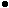1.1.6. General and Special Relativity

The early 20th century brought a number of profound developments on the observational and theoretical fronts which once again necessitated a fundamental revision to the accepted cosmological framework. To begin with, a refinement of Newtonian mechanics was required, and this was offered by Einstein in the form of the General Theory of Relativity. Although the theory is best understood in equation form, (see for instance Weinberg 1972; Coles and Lucchin 1995) two of the most important ideas of general relativity theory can be summarized as follows:Energy and Mass are equivalent. Any object which has energy also has mass and is therefore affected by gravity. Mass can be converted into energy and energy into mass. This discovery could now account for the enormous energy source that powered the stars and which could render them visible to us over distances of thousands of light years.Gravity is the manifestation of the curvature of space-time. That is, space communicates with matter and instructs it how to move and, in turn, matter communicates with space and instructs it how to curve.

In addition to General Relativity, Einstein also developed the case of Special Relativity which asserts that the laws of physics are identical in all inertial frames. For this to be true, the speed of light cannot be different in different frames, even if those frames are in relative motion. This condition is met if the speed of light is a Universal constant. This finite communication time, which was not a component of Newton's description of gravity, allows for cause and effect relations which leads to the concept of Causality. Among many things, special relativity shows for objects moving at high velocity time runs slower, lengths become shorter and masses increase. This last effect can be understood through relativity as a relation between the increase of kinetic energy in one frame and a mass increase in another. This leads to the important principle of equivalence, E= mc2. The conditions of general relativity follow from those of special relativity but are applied to an accelerating frame (such as a gravitational potential). Hence inertial and gravitational forces are the same phenomenon and there is an identity between inertial masses as derived from Newton's laws of motion and gravitational masses which produce the force.

The basic prediction is that Newtonian gravity is very accurate when the gravitational field is weak (meaning that space is locally flat) but breaks down when the gravitational field is very strong (meaning space is locally curved). This was first manifested observationally when precision measures of the orbit of Mercury disagreed with Newtonian mechanics. The resolution is provided by General Relativity. Mercury is sufficiently close to the Sun that it orbits in curved space and hence Newtonian mechanics provides an incomplete specification of its orbital parameters. The precession of the perihelion of Mercury's orbit and the bending of star light when it passes near the Sun are in excellent agreement with predictions from General Relativity and serve as its best verification.